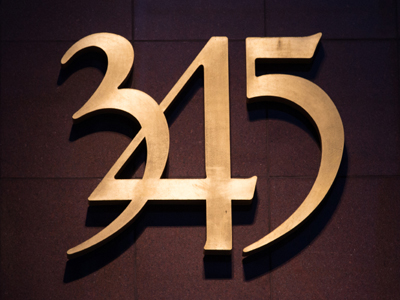345 is written three hundred and forty-five.

# Place Value 1

This Math quiz is called 'Place Value 1' and it has been written by teachers to help you if you are studying the subject at elementary school. Playing educational quizzes is an enjoyable way to learn if you are in the 3rd, 4th or 5th grade - aged 8 to 11.

It costs only \$12.50 per month to play this quiz and over 3,500 others that help you with your school work. You can subscribe on the page at Join Us

Place value works by using hundreds, tens, units, tenths and hundredths. You will have no doubt learned about place value in your math lessons. How a 2 can be worth 2, 20 or 200 depending on whether it is in the units, tens or hundreds column in a number. This quiz will test you on what you can remember.

1.
How many digits are there in our number system?
8
9
10
11
The ten digits are 0, 1, 2, 3, 4, 5, 6, 7, 8 and 9
2.
What does the 3 represent in the number 396?
3
30
300
3,000
As the three is in the hundreds column it represents 3 hundreds
3.
What is this number in figures - two hundred and one?
201
2,001
21
211
201 has 2 hundreds, 0 tens and 1 unit
4.
When dealing with place value what is the name for the ones?
Tens
Hundreds
Units
Thousands
Units and ones mean exactly the same in Maths
5.
What is this number in figures - four hundred and seventy one?
4,071
400,701
4,710
471
471 has 4 hundreds, 7 tens and 1 unit
6.
What is this number in words - 359?
Three thousand, five hundred and nine
Three hundred and fifty nine
Thirty five hundred and nine
Three thousand and fifty nine
359 has 3 hundreds, 5 tens and 9 units
7.
What does the 2 represent in the number 824?
2
20
200
2,000
A 2 in the tens column means 2 x 10 which is 20
8.
What is this number in words - 407?
Four thousand and seven
Four hundred and seventy
Four hundred and seven
Forty seven
407 has 4 hundreds, 0 tens and 7 units
9.
What does the 8 represent in the number 804?
8
80
800
8,000
An 8 in the hundreds column = 8 x 100 which is 800
10.
What does the 0 represent in the number 906?
0 tens
10
100
1,000
The zero in the ten column means there are no tens in the number but the zero still holds the ten's place
Author:  Amanda Swift